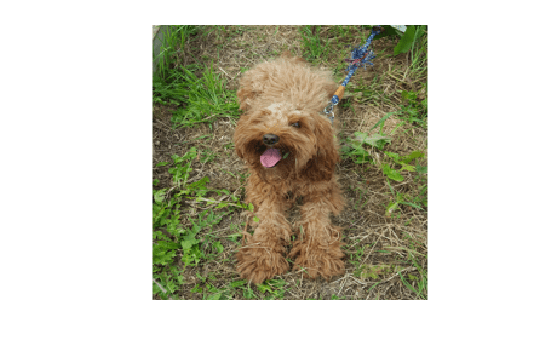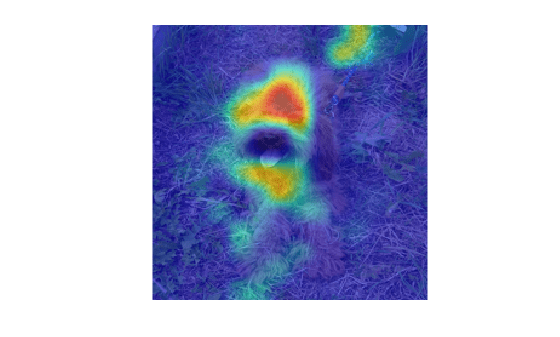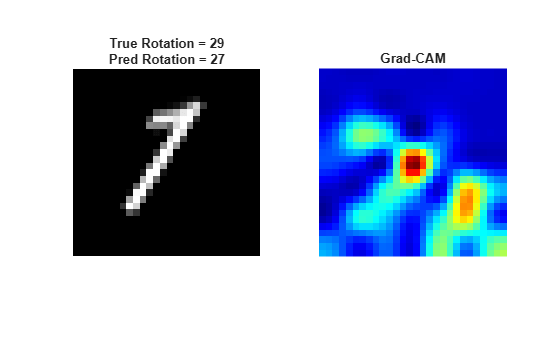## Syntax

``scoreMap = gradCAM(net,X,label)``
``scoreMap = gradCAM(net,X,reductionFcn)``
``[scoreMap,featureLayer,reductionLayer] = gradCAM(___)``
``___ = gradCAM(___,Name,Value)``

## Description

example

````scoreMap = gradCAM(net,X,label)` returns the gradient-weighted class activation mapping (Grad-CAM) map of the change in the classification score of image `X`, when the network `net` evaluates the class score for the class given by `label`. Use this function to explain network predictions and check that your network is focusing on the right parts of an image. The Grad-CAM interpretability technique uses the gradients of the classification score with respect to the final convolutional feature map. The parts of an image with a large value for the Grad-CAM map are those that most impact the network score for that class.Use this syntax to compute the Grad-CAM map for image or pixel classification tasks.```

example

````scoreMap = gradCAM(net,X,reductionFcn)` returns the Grad-CAM importance map using a reduction function. `reductionFcn` is a function handle that reduces the output activations of the reduction layer to a scalar value. This scalar fulfills the role of the class score for classification tasks, and generalizes the Grad-CAM technique to nonclassification tasks, such as regression. The `gradCAM` function computes the Grad-CAM map by differentiating the reduced output of the reduction layer with respect to the features in the feature layer. `gradCAM` automatically selects reduction and feature layers to use when computing the map. To specify these layers, use the `'ReductionLayer'` and `'FeatureLayer'` name-value arguments. Use this syntax to compute the Grad-CAM map for nonclassification tasks.```
````[scoreMap,featureLayer,reductionLayer] = gradCAM(___)` also returns the names of the feature layer and reduction layer used to compute the Grad-CAM map. Use this syntax with any of the input-argument combinations in previous syntaxes. ```

example

````___ = gradCAM(___,Name,Value)` specifies options using one or more name-value arguments in addition to the input arguments in previous syntaxes. For example, `'ReductionLayer','prob'` sets the reduction layer to the `net` layer named `'prob'`. ```

## Examples

collapse all

Use `gradCAM` to visualize which parts of an image are important to the classification decision of a network.

Import the pretrained network SqueezeNet.

`net = squeezenet;`

Import the image and resize it to match the input size for the network.

```X = imread("laika_grass.jpg"); inputSize = net.Layers(1).InputSize(1:2); X = imresize(X,inputSize);```

Display the image.

`imshow(X)`Classify the image to get the class label.

`label = classify(net,X)`
```label = categorical toy poodle ```

Use `gradCAM` to determine which parts of the image are important to the classification result.

`scoreMap = gradCAM(net,X,label);`

Plot the result over the original image with transparency to see which areas of the image contribute most to the classification score.

```figure imshow(X) hold on imagesc(scoreMap,'AlphaData',0.5) colormap jet```The network focuses predominantly on the back of the dog to make the classification decision.

Use Grad-CAM to visualize which parts of an image are most important to the predictions of an image regression network.

Load the sample data, which consists of synthetic images of handwritten digits. The third output contains the corresponding angles of rotation of the digits, in degrees.

```rng default [XTrain,~,YTrain] = digitTrain4DArrayData; [XTest,~,YTest] = digitTest4DArrayData; numTrainImages = numel(YTrain); idx = randperm(numTrainImages,20);```

Construct an image regression network that can predict the rotation of an image.

```layers = [ ... imageInputLayer([28 28 1],'Name','input') convolution2dLayer(12,25,'Name','conv') reluLayer('Name','relu') fullyConnectedLayer(1,'Name','fc') regressionLayer('Name','output')];```

Specify the training options.

```options = trainingOptions('sgdm', ... 'InitialLearnRate',0.001, ... 'Verbose',false, ... 'Plots','training-progress');```

Train the network.

`net = trainNetwork(XTrain,YTrain,layers,options);`Evaluate the performance of the network on a test image.

`testDigit = XTest(:,:,:,idx(4));`

Use `predict` to predict the angle of rotation and compare the predicted rotation to the true rotation.

`predRotation = predict(net,testDigit)`
```predRotation = single -47.5497 ```
`trueRotation = YTest(idx(4))`
```trueRotation = -40 ```

Visualize the regions of the image most important to the network prediction using `gradCAM`. Select the ReLU layer as the feature layer and the fully connected layer as the reduction layer.

```featureLayer = 'relu'; reductionLayer = 'fc';```

Define the reduction function. The reduction function must reduce the output of the reduction layer to a scalar value. The Grad-CAM map displays the importance of different parts of the image to that scalar. In this regression problem, the network predicts the angle of rotation of the image. Therefore, the output of the fully connected layer is already a scalar value and so the reduction function is just the identity function.

`reductionFcn = @(x)x;`

```scoreMap = gradCAM(net,testDigit,reductionFcn, ... 'ReductionLayer',reductionLayer, ... 'FeatureLayer',featureLayer);```

Display the Grad-CAM map over the test image.

```ax(1) = subplot(1,2,1); imshow(testDigit) title("True Rotation = " + trueRotation + '\newline Pred Rotation = ' + round(predRotation,0)) colormap(ax(1),'gray') ax(2) = subplot(1,2,2); imshow(testDigit) hold on imagesc(scoreMap) colormap(ax(2),'jet') title("GradCAM") hold off```The Grad-CAM map shows that the network is focusing on the area in the bottom left, which is where the tail of the digit would be if the image had zero rotation. The map suggests that to predict the negative rotation, the network is using the empty space.

## Input Arguments

collapse all

Trained network, specified as a `SeriesNetwork`, `DAGNetwork`, or `dlnetwork` object. You can get a trained network by importing a pretrained network or by training your own network using the `trainNetwork` function or custom training. For more information about pretrained networks, see Pretrained Deep Neural Networks.

`net` must contain a single input layer and a single output layer. The input layer of `net` must be an `imageInputLayer` or an `image3dInputLayer`.

Input data, specified as a numeric array or formatted `dlarray` object.

`X` must have size equal to the input size of the network.

Data Types: `single` | `double` | `int8` | `int16` | `int32` | `int64` | `uint8` | `uint16` | `uint32` | `uint64`

Class label to use for calculating the Grad-CAM map for image classification and semantic segmentation tasks, specified as a categorical, a character vector, a string scalar, a numeric index, or a vector of these values.

For `dlnetwork` objects, you must specify `label` as a categorical or a numeric index.

If you specify `label` as a vector, the software calculates the feature importance for each class label independently. In that case, `scoreMap(:,:,k)` corresponds to the map for `label(k)`.

The `gradCAM` function sums the spatial dimensions of the reduction layer for class `label`. Therefore, you can specify `label` as the classes of interest for semantic segmentation tasks and `gradCAM` returns the Grad-CAM importance for each pixel.

Example: `["cat" "dog"]`

Example: `[1 5]`

Data Types: `char` | `string` | `categorical`

Reduction function, specified as a function handle. The reduction function reduces the output activations of the reduction layer to a single value and must reduce a `dlarray` object to a `dlarray` scalar. This scalar fulfills the role of `label` in classification tasks, and generalizes the Grad-CAM technique to nonclassification tasks, such as regression.

Grad-CAM uses the reduced output activations of the reduction layer to compute the gradients for the importance map.

Example: `@x(x)`

Data Types: `function_handle`

### Name-Value Arguments

Specify optional pairs of arguments as `Name1=Value1,...,NameN=ValueN`, where `Name` is the argument name and `Value` is the corresponding value. Name-value arguments must appear after other arguments, but the order of the pairs does not matter.

Before R2021a, use commas to separate each name and value, and enclose `Name` in quotes.

Example: `'FeatureLayer','conv10','ReductionLayer','prob','OutputUpsampling','bicubic','ExecutionEnvironment','gpu'` computes the Grad-CAM map with respect to layers `'conv10'` and `'prob'`, executes the calculation on the GPU, and upsamples the resulting map to the same size as the input image using bicubic interpolation.

Name of the feature layer to extract the feature map from when computing the Grad-CAM map, specified as a string or character vector. For most tasks, use the last ReLU layer with nonsingleton spatial dimensions or the last layer that gathers the outputs of ReLU layers (such as depth concatenation or addition layers). If your network does not contain any ReLU layers, specify the name of the final convolutional layer that has nonsingleton spatial dimensions in the output.

The default value is the final layer with nonsingleton spatial dimensions. Use the `analyzeNetwork` function to examine your network and select the correct layer.

Example: `'FeatureLayer','conv10'`

Data Types: `char` | `string`

Name of the reduction layer to extract output activations from when computing the Grad-CAM map, specified as a string or character vector. For classification tasks, this layer is usually the final softmax layer. For other tasks, this layer is usually the penultimate layer for DAG and series networks and the final layer for `dlnetwork` objects.

The default value is the penultimate layer in DAG and series networks, and the final layer in `dlnetwork` objects. Use the `analyzeNetwork` function to examine your network and select the correct layer.

Example: `'ReductionLayer','prob'`

Data Types: `char` | `string`

Data format assigning a label to each dimension of the input data, specified as a character vector or a string. Each character in the format must be one of the following dimension labels:

• `S` — Spatial

• `C` — Channel

• `B` — Batch

For more information, see `dlarray`.

Example: `'Format','SSC'`

Data Types: `char` | `string`

Output upsampling method, specified as the comma-separated pair consisting of` 'OutputUpsampling'` and one of the following values:

• `'bicubic'` — Use bicubic interpolation to produce a smooth map the same size as the input data.

• `'nearest'` — Use nearest-neighbor interpolation to expand the map to the same size as the input data.

• `'none'` — Use no upsampling. The map can be smaller than the input data.

If `'OutputUpsampling'` is `'nearest'` or `'bicubic'`, the computed map is upsampled to the size of the input data using the `imresize` function for 2-D data and the `imresize3` (Image Processing Toolbox) function for 3-D data. For 3-D data, the option `'bicubic'` uses `imresize3` with the `'cubic'` method.

Example: `'OutputUpsampling','bicubic'`

Hardware resource for computing the map, specified as the comma-separated pair consisting of `'ExecutionEnvironment'` and one of the following.

• `'auto'` — Use the GPU if one is available. Otherwise, use the CPU.

• `'cpu'` — Use the CPU.

• `'gpu'` — Use the GPU.

The GPU option requires Parallel Computing Toolbox™. To use a GPU for deep learning, you must also have a supported GPU device. For information on supported devices, see GPU Support by Release (Parallel Computing Toolbox). If you choose the `'gpu'` option and Parallel Computing Toolbox and a suitable GPU are not available, then the software returns an error.

Example: `'ExecutionEnvironment','gpu'`

## Output Arguments

collapse all

Grad-CAM importance map, returned as a numeric matrix or a numeric array. Areas in the map with higher positive values correspond to regions of input data that contribute positively to the prediction.

• For classification tasks, `scoreMap` is the gradient of the final classification score for the specified class, with respect to each feature in the feature layer.

• For other types of tasks, `scoreMap` is the gradient of the reduced output of the reduction layer, with respect to each feature in the feature layer.

`scoreMap(i,j)` corresponds to the Grad-CAM importance at the spatial location `(i,j)`. If you provide `label` as a vector of categoricals, character vectors, or strings, then `scoreMap(:,:,k)` corresponds to the map for `label(k)`.

Name of the feature layer to extract the feature map from when computing the Grad-CAM map, returned as a string.

By default, `gradCAM` chooses a feature layer to use to compute the Grad-CAM map. This layer is the final layer with nonsingleton spatial dimensions. You can specify which feature layer to use using the `'FeatureLayer'` name-value argument. When you specify the `'FeatureLayer'` name-value argument, `featureLayer` returns the same value.

Name of the reduction layer to extract output activations from when computing the Grad-CAM map, returned as a string.

By default, `gradCAM` chooses a reduction layer to use to compute the Grad-CAM map. This layer is the penultimate layer in DAG and series networks, and the final layer in `dlnetwork` objects. You can also specify which reduction layer to use using the `'ReductionLayer'` name-value argument. When you specify the `'ReductionLayer'` name-value argument, `reductionLayer` returns the same value.

collapse all

Gradient-weighted class activation mapping (Grad-CAM) is an explainability technique that can be used to help understand the predictions made by a deep neural network . Grad-CAM, a generalization of the CAM technique, determines the importance of each neuron in a network prediction by considering the gradients of the target flowing through the deep network.

Grad-CAM computes the gradient of a differentiable output, for example class score, with respect to the convolutional features in the chosen layer. The gradients are spatially pooled to find the neuron importance weights. These weights are then used to linearly combine the activation maps and determine which features are most important to the prediction.

Suppose you have an image classification network with output yc, representing the score for class c, and want to compute the Grad-CAM map for a convolutional layer with k feature maps (channels), Aki,j, where i,j indexes the pixels. The neuron importance weight is

where N is the total number of pixels in the feature map. The Grad-CAM map is then a weighted combination of the feature maps with an applied ReLU:

`$M=\text{ReLU}\left({\sum }_{k}{\alpha }_{k}^{c}{A}^{k}\right).$`

The ReLU activation ensures you get only the features that have a positive contribution to the class of interest. The output is therefore a heatmap for the specified class, which is the same size as the feature map. The Grad-CAM map is then upsampled to the size of the input data.

Although Grad-CAM is commonly used for image classification tasks, you can compute a Grad-CAM map for any differentiable activation. For example, for semantic segmentation tasks, you can calculate the Grad-CAM map by replacing yc with ${\sum }_{\left(i,j\right)\in S}{y}_{ij}^{c}$, where S is the set of pixels of interest and yi,jc is 1 if pixel (i,j) is predicted to be class c, and 0 otherwise . You can use the `gradCAM` function for nonclassification tasks by specifying a suitable reduction function that reduces the output activations of the reduction layer to a single value and takes the place of yc in the neuron importance weight equation.

## Tips

• The `reductionFcn` function receives the output from the reduction layer as a traced `dlarray` object. The function must reduce this output to a scalar `dlarray`, which `gradCAM` then differentiates with respect to the activations of the feature layer. For example, to compute the Grad-CAM map for channel 208 of the softmax activations of a network, the reduction function is `@(x)(x(208))`. This function receives the activations and extracts the 208th channel.

• The `gradCAM` function automatically chooses reduction and feature layers to use when computing the Grad-CAM map. For some networks, the chosen layers might not be suitable. For example, if your network has multiple layers that can be used as the feature layer, then the function chooses one of those layers, but its choice might not be the most suitable. For such networks, specify which feature layer to use using the `'FeatureLayer'` name-value argument.

 Selvaraju, Ramprasaath R., Michael Cogswell, Abhishek Das, Ramakrishna Vedantam, Devi Parikh, and Dhruv Batra. “Grad-CAM: Visual Explanations from Deep Networks via Gradient-Based Localization.” 2017 (October 2017): 618–626, https://doi.org/10.1109/ICCV.2017.74.

 Vinogradova, Kira, Alexandr Dibrov, and Gene Myers. “Towards Interpretable Semantic Segmentation via Gradient-Weighted Class Activation Mapping.” Proceedings of the AAAI Conference on Artificial Intelligence 34, no. 10 (April 2020): 13943–13944, https://doi.org/10.1609/aaai.v34i10.7244.